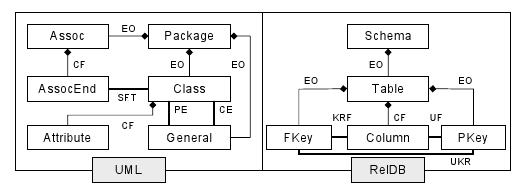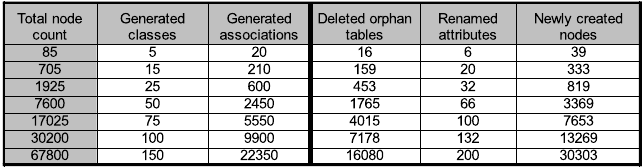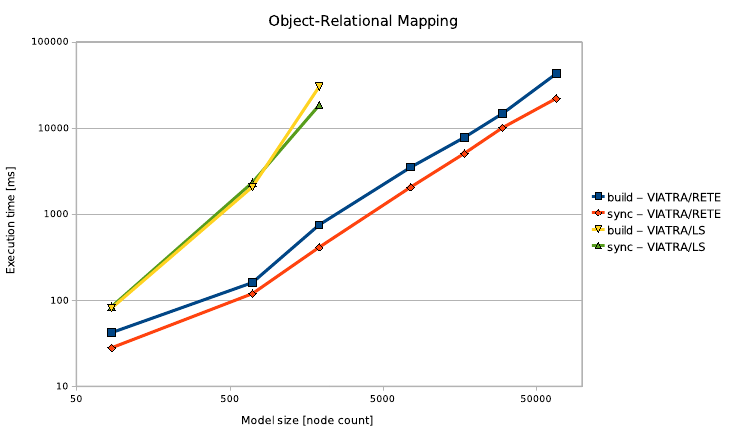# VIATRA2/ORM benchmark

(diff) ← Older revision | Latest revision (diff) | Newer revision → (diff)

## Outdated page

The currently maintained wiki is available at http://wiki.eclipse.org/VIATRA

## Object-relational mapping benchmark (field: model synchronisation)

### Benchmark description

The Object-to-Relational schema mapping (ORM) benchmark, as presented here, is an extension of the original benchmark proposed in Sec.4 of:

```Varró G., Schürr, A., Varró D.: Benchmarking for graph transformation. In: Proc. IEEE
Symposium on Visual Languages and Human-Centric Computing (VL/HCC 05), Dallas,
Texas, USA, IEEE Press (September 2005) 79–88
```

The original transformation processed UML class diagrams to produce corresponding relational database schemata, according to the known mapping rules. Since a straightforward application of the incremental pattern matching approach is the synchronization between source and target models, we extended the benchmark by two additional sequences: (i) after the initial mappings are created, the source models are modified, and, in an additional pass, (ii) the system has to synchronize the changes to the target model (i.e. find the changes in the source and alter the target accordingly). A local search-based algorithm has to search for the changes first, while an incremental pattern matcher can track changes in the source model so that the model parts affected are instantly available for the synchronization sequence.

Execution phases:

1. The generation phase creates the model graph.
2. The build phase maps creates the initial mapping of the UML model into the relational schema domain, with reference models connecting mapped model objects.
3. The modification phase modifies the UML models programmatically to emulate user editing actions.
4. Finally, the synchronization phase locates the affected model elements and makes changes in the schema model accordingly.

### MetamodelUML and DB metamodel (classname attribute omitted).

The UML and DB metamodels follow the original benchmark proposition (with minor changes in naming). Our reference metamodel is somewhat more refined, but still relies on simple edges instead of the more versatile approach of reference entities.

```entity(UML)
{
entity(model);
entity(metamodel) {

entity(class) {
entity(attribute);
relation(cl2attrs, class, attribute);
isAggregation(cl2attrs, true);
relation(name, class, datatypes.String);
}
entity(assoc) {
entity(end);
relation(assoc2ends, assoc, end);
isAggregation(assoc2ends, true);
relation(end2cl, end, class);
relation(name, assoc, datatypes.String);
}
entity(general) {
relation(parent, general, class);
relation(child, general, class);
}
entity(package) {
relation(p2as, package, assoc);
isAggregation(p2as, true);
relation(p2cls, package, class);
isAggregation(p2cls, true);
relation(p2gs, package, general);
isAggregation(p2gs, true);
}
}

}
entity ( DB ) {
entity(model);
entity(metamodel) {
entity ( schema ) {
relation (tables, schema, table);
isAggregation(tables, true);
}
entity ( table ) {
relation ( t2pkey , table , pKey );
isAggregation(t2pkey, true);
relation ( t2cols , table , column );
isAggregation(t2cols, true);
relation ( t2fkeys , table , fKey );
isAggregation(t2fkeys, true);
relation(name, table, datatypes.String);
}
entity ( column );
entity ( fKey ) {
relation ( cref , fKey , column );
relation ( ukr , fKey , pKey );
}
entity ( pKey ) {
relation ( pkey2col , pKey , column );
}
}
}

// reference model
relation(schemaRef, UML.metamodel.package, DB.metamodel.schema);
relation(tableRef, UML.metamodel.class, DB.metamodel.table);
relation(tableRef, UML.metamodel.assoc, DB.metamodel.table);
relation(fKeyRef, UML.metamodel.assoc.end, DB.metamodel.fKey);
relation(fKeyRef, UML.metamodel.general, DB.metamodel.fKey);
relation(colRef, UML.metamodel.class.attribute, DB.metamodel.column);```

### Initial model: test case generation

In order to produce sufficiently large model graphs for the measurements, we implemented a simple generator as described in the referenced article. By this approach, a fully connected graph is created, i.e. for N UML classes, N(N − 1) directed associations are defined (with each association represented as three nodes – an association node and two endpoints). Additionally, each UML class can reference K attributes, thus, for a given N and K, N + 3N(N − 1) + NK nodes and 4N(N − 1) + NK edges are created (see the figure on the side). Although the model produced is not “realistic” in the sense that very few practical UML class diagrams are fully connected, the method is quite efficient in creating large graphs quickly.

### Transformation details

The build phase follows the original Varró benchmark description. Here we give the implementation of a representative graph transformation rule.

```pattern classRule_lhs(P, C, S, Str) =
{
package(P);
class(C);
package.p2cls(P2C, P, C);
schema(S);
package.schemaRef(R1, P, S);
datatypes.String(Str);
class.name(N1, C, Str);
neg pattern mapped(C, TN, REFN) = {
class(C);
table(TN);
class.tableRef(REFN, C, TN);
}
}
rule classRule(in C, in S, in Str,
out T, out TPK, out TID, out R2, out EO2, out EO3, out CF, out UF, out N2) = seq{
new (table(T) in S);
rename(T,name(C));
new (class.tableRef(R2, C, T));
rename(R2,"class_table");
new (schema.tables(EO2, S, T));
rename(EO2,"_"+name(T));
new (table.name(N2, T, Str));
rename(N2,"table_name");
new (pKey(TPK) in T);
rename(TPK,"primaryKey");
new (table.t2pkey(EO3, T, TPK));
rename(EO3,"table_pkey");
new (column(TID) in T);
rename(TID,"id");
new (table.t2cols(CF, T, TID));
rename(CF,"_"+name(TID));
new (pKey.pkey2col(UF, TPK, TID));
rename(UF,"pkey_column");
}```

To support incremental synchronisation between source and target models, to avoid rebuilding target models in each pass, the reference model is used to establish a mapping relationship between source and corresponding target model elements. With correspondence edges, it is possible to track changes in both the source and target models: for instance, the graph pattern featured below matches tables in the schema model which are no longer referenced by classes or associations in the UML models (orphan tables).

Renaming (value changing) may be expressed e.g. by matching for both the attribute and the mapped column, and looking for pairs where the name (attribute value) is different. The modification sequence results in the following synchronization sequence:

1. all orphan tables belonging to the deleted classes and their associations are deleted;
2. all orphan tables belonging to the deleted associations are deleted;
3. column names mapped to the renamed attributes are changed;
4. new tables are added for the newly created class and the new associations.

The following representative pattern identifies orphan DB tables that have no reason anymore to exist, and shall be deleted:

```pattern orphanTable(T) =
{
table(T);
neg pattern mapped(T) =
{
class(C);
table(T);
class.tableRef(REFN, C, T);
} or {
assoc(A);
table(T);
assoc.tableRef(REFN, A, T);
}
}```

### Measurement resultsModel and synchronization sequence sizes for the ORM benchmark

The measurements reported here have been carried out on a standard desktop computer with a 2 GHz Intel Core2 processor with 2 gigabytes of system RAM available, running version 1.6.0-05 of the 32-bit Sun Java SE Runtime. In general, ten test runs were executed, and the results were calculated by averaging the values excluding the highest and lowest number. The transformation sequences were coded so that little or no output was generated; in the case of VIATRA 2, we refrained from disabling the GUI. Execution times were measured with millisecond precision as allowed by the operating system calls.

The ORM synchronization benchmark was executed with the VIATRA 2 tool (due to time constraints, measurements with GrGEN.NET and others are left as future work). Models up to 67800 nodes (with edges, the total model size is 157800 model elements) were generated (see the table to the right) and the execution time for the build and synchronization phases was measured.Results for the ORM synchronization benchmark

The results are shown in the figure to the right (model size is the total number of nodes). It is gain revealed that the scaling characteristic of both phases is exponential for VIATRA/LS and linear for VIATRA/RETE. With respect to synchronization, the constant difference between the build and sync phases for VIATRA/RETE means a constant multiplier; thus, since the model elements affected by the modification sequence are a linear fraction of the whole model, it can be concluded that the execution time for the synchronization process is a linear function of the model elements affected (as expected), and independent of the size of the rest of the model. VIATRA/LS, on the other hand, exhibits an ever increasing time difference between build and sync, thus, the time taken for the synchronization process increases exponentially with the number of affected model elements (again, as expected, since in the case of local search, the system has to locate the changed elements ﬁrst which is an additional graph traversal). It is important to note that for “practical” model sizes (e.g. below the 5000 node count range), VIATRA/RETE can perform a synchronization affecting a considerable portion of the model in the 10-500 msec range which makes the approach very suitable for interactive applications.

In addition to execution times, the memory consumed by the Java Virtual Machine was also recorded. The sequence for the RETE matcher (75, 100, 114, 245, 490, 750, 1000 megabytes respectively for model sizes from 85 to 67800 nodes) shows a linearly expanding RETE network as the node count grows, which is in-line with our expectations based on the nature of the RETE building algorithm (note that the above ﬁgures include the whole user interface with a complete Eclipse instance).